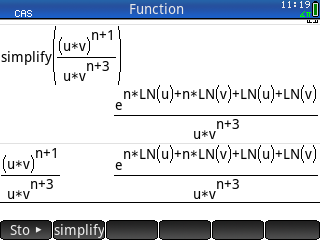Simplifying Rational Expressions
07-08-2021, 08:28 AM
Post: #1saschaJunior Member Posts: 9 Joined: Jun 2021
Simplifying Rational Expressions
How can I simplify the expression below with the HP Prime? I like to see u^n over v^2 as the result.Casio fx-CG500, HP Prime 2AP18AA
07-08-2021, 09:18 AM
Post: #2
 rawiMember Posts: 132 Joined: Nov 2019
RE: Simplifying Rational Expressions
If I make
simplify((u^(n+1)*v^(n+1))/(u*v^(n+3)))
I get a little bit further to u^(n+1)/(u*v^2), but it is not able to reduce the fraction to u^n/v^2. If I try to simplify the exptression u^(n+1)/(u*v^2) I get what you already got.

BTW: If I try expand(((u*v)^(n+1))/(u*v^(n+3))) with the TI89 Titanium I get (u*v)^n/v^(n+2).
If I do expand((u^(n+1)*v^(n+1))/(u*v^(n+3))) I get u^n/v^2.
So this is not perfect but better than the Prime.

Result with TI nspire CX II-T CAS:
expand(((u*v)^(n+1))/(u*v^(n+3))) --> (u*v)^n/(u*v^(n+3))
expand((u^(n+1)*v^(n+1))/(u*v^(n+3))) --> u^n/v^2

So it seams that the brackets in the numerator make things more difficult.

It's really strange because it is mathematically so simple.

Best

Raimund
07-08-2021, 09:38 AM
Post: #3
 robmioMember Posts: 165 Joined: Jan 2020
RE: Simplifying Rational Expressions
Try to use, in sequence, "texpand" and then "simplify"

Greetings, Robmio

Attached File(s) Thumbnail(s)07-08-2021, 09:58 AM (This post was last modified: 07-08-2021 09:59 AM by sascha.)
Post: #4saschaJunior Member Posts: 9 Joined: Jun 2021
RE: Simplifying Rational Expressions
(07-08-2021 09:38 AM)robmio Wrote:  Try to use, in sequence, "texpand" and then "simplify"

Greetings, Robmio

That's what I get:Casio fx-CG500, HP Prime 2AP18AA
07-08-2021, 10:26 AM
Post: #5
 robmioMember Posts: 165 Joined: Jan 2020
RE: Simplifying Rational Expressions
Hi, you don't have to use "expand", but "texpand", followed by "simplify".

Attached File(s) Thumbnail(s)07-08-2021, 11:59 AM
Post: #6
 Albert ChanSenior Member Posts: 2,142 Joined: Jul 2018
RE: Simplifying Rational Expressions
CAS> simplify(pow2exp((u*v)^(n+1)/(u*v^(n+3)))) ﻿ ﻿ ﻿ ﻿ ﻿ ﻿ ﻿ ﻿ ﻿ ﻿ ﻿ ﻿ ﻿ ﻿ → u^n/v^2
CAS> simplify(pow2exp(u^(n+1)*v^(n+1)/(u*v^(n+3)))) ﻿ ﻿ ﻿ ﻿ ﻿ ﻿ → u^n/v^2
07-08-2021, 12:18 PM
Post: #7saschaJunior Member Posts: 9 Joined: Jun 2021
RE: Simplifying Rational Expressions
(07-08-2021 10:26 AM)robmio Wrote:  Hi, you don't have to use "expand", but "texpand", followed by "simplify".

Thanks! Amazing, this works:Casio fx-CG500, HP Prime 2AP18AA
07-08-2021, 12:23 PM
Post: #8saschaJunior Member Posts: 9 Joined: Jun 2021
RE: Simplifying Rational Expressions
(07-08-2021 11:59 AM)Albert Chan Wrote:  CAS> simplify(pow2exp((u*v)^(n+1)/(u*v^(n+3)))) ﻿ ﻿ ﻿ ﻿ ﻿ ﻿ ﻿ ﻿ ﻿ ﻿ ﻿ ﻿ ﻿ ﻿ → u^n/v^2
CAS> simplify(pow2exp(u^(n+1)*v^(n+1)/(u*v^(n+3)))) ﻿ ﻿ ﻿ ﻿ ﻿ ﻿ → u^n/v^2

This works great. Thanks!Casio fx-CG500, HP Prime 2AP18AA
07-09-2021, 03:11 PM (This post was last modified: 07-09-2021 07:25 PM by Hlib.)
Post: #9HlibMember Posts: 242 Joined: Jan 2015
RE: Simplifying Rational Expressions
My afx-2.0 calculator outputs:
simplify ((U×V)^(N+1)/((U×V)^(N+3)))
result is 1/(U^2×V^2)
Is this correct?
EDIT
My input mistake:
(u×v)^(n+1)/(u×v^(n+3))
Yes, Prime wins.
07-09-2021, 07:07 PM
Post: #10
 dah145Junior Member Posts: 37 Joined: Aug 2020
RE: Simplifying Rational Expressions
(07-09-2021 03:11 PM)Hlib Wrote:  My afx-2.0 calculator outputs:
simplify ((U×V)^(N+1)/((U×V)^(N+3)))
result is 1/(U^2×V^2)
Is this correct?

The original expression is ((U*V)^(N+1))/(U*(V^(N+3)), my algebra FX 2.0 isn't able to simplify it.
07-09-2021, 07:27 PM
Post: #11HlibMember Posts: 242 Joined: Jan 2015
RE: Simplifying Rational Expressions
Yes, I have edit my mistake.
 « Next Oldest | Next Newest »

User(s) browsing this thread: 1 Guest(s)